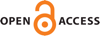Download this articleFor screen For printingRecent IssuesThe Journal About the Journal Editorial Board Editors’ Interests Subscriptions Submission Guidelines Submission Form Policies for Authors Ethics Statement ISSN: 1948-206X (e-only) ISSN: 2157-5045 (print) Author Index To Appear Other MSP JournalsSharp pointwise and uniform estimates for $\bar\partial$

### Robert Xin Dong, Song-Ying Li and John N. Treuer

Vol. 16 (2023), No. 2, 407–431##### Abstract

We use weighted ${L}^{2}$-methods to obtain sharp pointwise estimates for the canonical solution to the equation $\overline{\partial }u=f$ on smoothly bounded strictly convex domains and the Cartan classical domains when $f$ is bounded in the Bergman metric $g$. We provide examples to show our pointwise estimates are sharp. In particular, we show that on the Cartan classical domains $\mathrm{\Omega }$ of rank $2$ the maximum blow-up order is greater than $-\mathrm{log}{\delta }_{\mathrm{\Omega }}\left(z\right)$, which was obtained for the unit ball case by Berndtsson. For example, for $\mathrm{\Omega }$ of type $\mathrm{IV}\left(n\right)$ with $n\ge 3$, the maximum blow-up order is $\delta {\left(z\right)}^{1-n∕2}$ because of the contribution of the Bergman kernel. Additionally, we obtain uniform estimates for the canonical solutions on the polydiscs, strictly pseudoconvex domains and the Cartan classical domains under stronger conditions on $f$.

##### Keywords
$L^2$ minimal solution, canonical solution, $\bar\partial$ equation, Cartan classical domain, Bergman kernel, Bergman metric
##### Mathematical Subject Classification
Primary: 32A25
Secondary: 32A36, 32M15, 32W05
##### Milestones